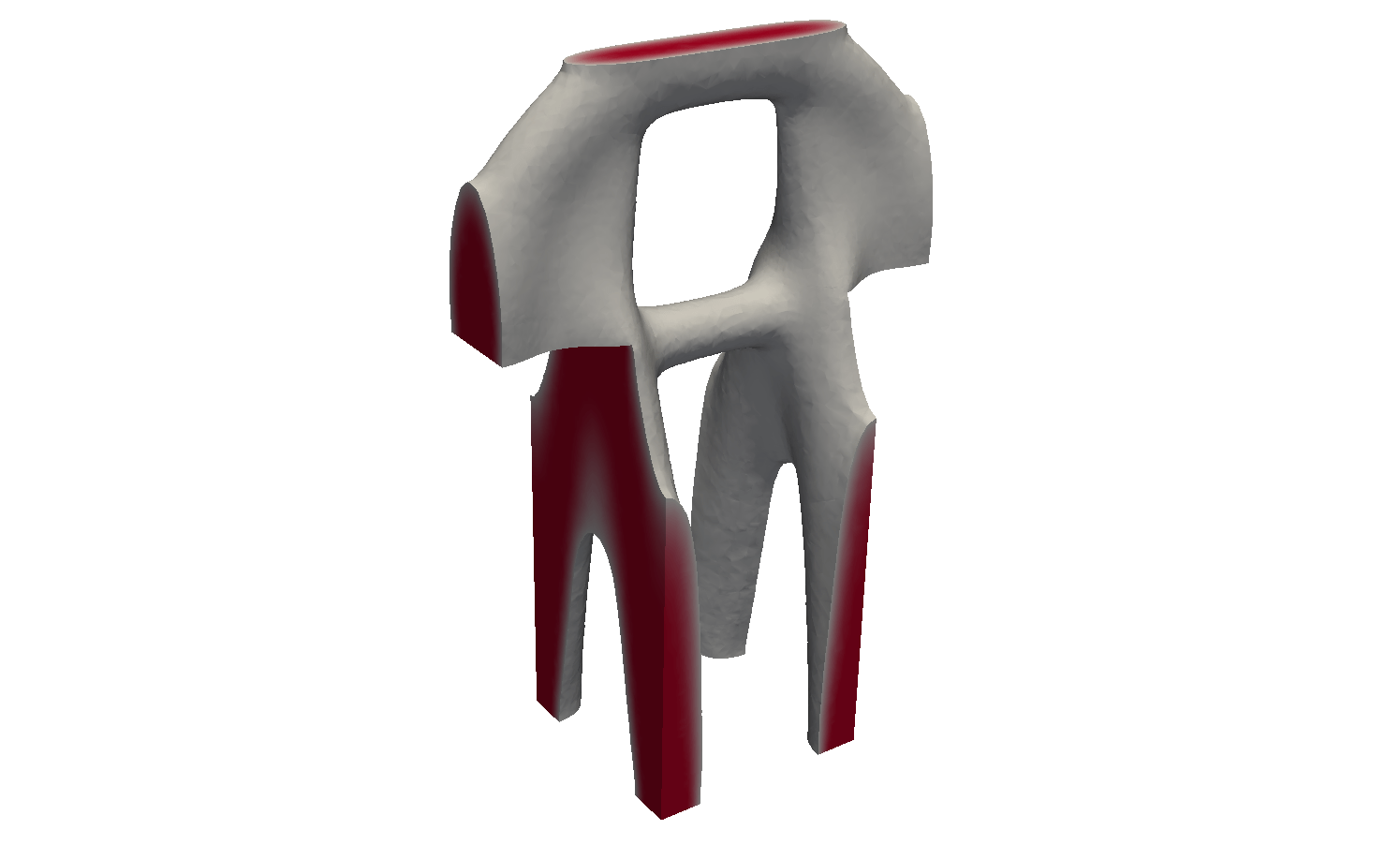# Topology Optimization and Risk ManagementEfficient design is one of the most important objectives in modern engineering. In a multitude of applications this requires the balancing of cost savings and risk management. In the design of load bearing structures it is the goal to minimize material usage and weight while still ensuring design limits and constraints. Less material in key components like the main frame assembly leads to additional savings in the support structures.

#### Model

Linear elasticity gives a prediction of the physical situation for a given workpiece subject to a known load. Here a shape is considered optimal when it minimizes the elastic energy. Hence, the compliance on the load boundary is chosen as the cost functional.

Phase-field approaches for topology optimization allow for implicit topology changes. This is a big advantage compared to parametric approaches where either the initial conditions provide a fixed topology it is altered explicitly by some algorithm. The extension of the model to include stochastic input data is straight forward. A proper risk measure like the conditional value at risk (CVaR) is used for the cost measure. Both material and applied load are modeled with uncertainties to account for production flaws and usage profiles of the work piece.

#### Simulation

An AllenCahn gradient flow approach leads to a pseudo time stepping algorithm realizing a steepest gradient descent. The resulting equations for the state, adjoint, and gradient are solved with standard finite element methods. Heuristics are used for the step with in the time stepping and for the local mesh quality. This is paramount for three dimensional applications as especially the elasticity equations lead to high computational costs. The stochastic integration is done with Monte Carlo approaches or tensor reconstructions. The latter allows for a faster and more robust approximation of rare events.

#### Examples

The first example mimics a load structure in two dimensions where the left end is fixed and the right side is support on a roller bearing. The load is applied to a small area in the middle of the lower bound. The deterministic solution for a straight downward pointing load is almost symmetrical.

The stochastic settings models varying load directions as well as local material weaknesses. Considering these load scenarios the mean solution of the optimization process is hard to interpret and does not give a good idea about a proper construction. The CVaR functional in place of the compliance functional is a proper risk measure and leads to a form which considers risk for a prescribed probability as well as compliance.setting, deterministic solution, mean of individual sample solutions, CVaR(0.8) solution

The second example models a cantilever situation in three dimensions where a load applied at the right side (full length, underneath) needs to be fixed to a wall (left end). The solution exhibits one hole and one counter support beam.solution of the cantilever example cutoff at 0.5

#### Preprint

M. Eigel, J. Neumann, R. Schneider, S. Wolf
Stochastic topology optimisation with hierarchical tensor reconstruction
WIAS Preprint 2362, 2016

#### Support

This MATHEON project is support by the Einstein Foundation Berlin through the Einstein Center for Mathematics Berlin (ECMath).

#### Contact

Dr. Johannes Neumann
Weierstrass Institute for Applied Analysis and Stochastics, Berlin

E-mail: johannes.neumann@wias-berlin.de
Website: http://wias-berlin.de/projects/ECMath-SE13/